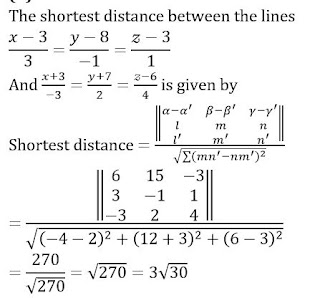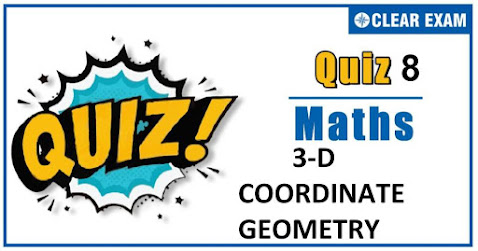## .question { margin-bottom: 20px; border-bottom: 1px solid #ddd; } .ques { font-size: 15px; font-weight: bold; line-height: 150%; } .q-options { padding: 0 !important; list-style: none; margin: 15px 0 !important; } .opt { font-size: 14px; font-weight: normal; } .answer { color: blue; display: none; padding-bottom: 15px; line-height: 150%; } .opt-right.active{ background: #a3ff91; font-size: 19px; line-height: 150%; border: 1px solid ##a3ff91; -webkit-border-radius: 5px; -moz-border-radius: 5px; border-radius: 5px; margin: 10px 0; } .mcq-info { margin: 20px 0 40px; color: #060553; line-height: 150%; } .timer{ overflow: hidden; margin: 20px 0; background: #f7f7f7; padding: 10px; border: 1px solid #eee; border-radius: 3px; } .start-btn, .time-btn, .stop-btn, #reset-start{ border: 1px solid #777; -o-border-radius: 2px; -ms-border-radius: 2px; -webkit-border-radius: 2px; border-radius: 2px; color: #fff; padding: 7px 15px; width: auto; height: auto; line-height: 150%; background: #d12F2F; cursor: pointer; margin-right: 10px; } #reset-start{ background: #0E2230; color: #fff; border-color: #0E2230; display: none; } .start-btn:hover, .time-btn:hover, .stop-btn:hover{ background: #0E2230; color: #fff; border-color: #0E2230; } #reset-start:hover{ background: #d12F2F; color: #fff; border-color: #d12F2F; } @media screen and (max-width: 767px){ .ques, .answer, .mcq-info, .opt{ font-size: 13px; } } Q1. If projection of a line on x,y and z-axes are 6,2 and 3 respectively, then direction cosines of the line is  (6/11,2/11.5/11)  (6/11,2/11.3/11)  (7/6,7/2,7/3)  (6/7,2/7,3/7) Solution Direction cosines =(6/√(36+4+9),2/√(36+4+9),3/√(36+4+9)) =(6/7,2/7,3/7) Q2.The shortest distance between the lines (x-3)/3=(y-8)/(-1)=(z-3)/1 and (x+3)/(-3)=(y+7)/2=(z-6)/4 is  5√30  3√30  2√30  √30 SolutionQ3.  The equation of the plane through the line of intersection of planes ax+by+cz+d=0,a^'x+b^'y+c^'z+d^'=0 and parallel to the line y=0,z=0 is   (ab^'-a'b)x+(bc^'-b'c)y+(ad^'-a'd)=0  (ab^'-a'b)x+(bc^'-b'c)y+(ad^'-a'd)z=0  None of these  (ab^'-a'b)y+(ac^'-a'c)z+(ad^'-a'd)=0 SolutionQ4. The vector equation of the plane passing through the origin and the line of intersection of the plane r ⃗∙a ⃗=λ and r ⃗∙b ⃗=μ is  r ⃗∙(λ a ⃗-μ b ⃗ )=0  r ⃗∙(λ a ⃗+μ b ⃗ )=0  r ⃗∙(λ b ⃗+μ a ⃗ )=0  r ⃗∙(λ b ⃗-μ a ⃗ )=0 SolutionQ5.The image (or reflection) of the point (1,2,-1) in the plane r ⃗∙(3i ̂-5j ̂+4k ̂ )=5 is  (73/25,-6/5,39/25)  (73/25,6/5,39/25)  (-1,-2,1)  None of these SolutionQ6. If the direction cosines of a line are (1/c,1/c,1/c), then  c=±√2  c=±√3 c>2  0 Solution Since,DC^' sof a line are(1/c,1/c,1/c) ∴ (1/c)^2+(1/c)^2+(1/c)^2=1 ⟹ c^2=3⟹c=± √3 Q7.The xy-plane divides the line joining the points (-1,3,4)and (2,-5,6)  Internally in the ratio 2 : 3  Internally in the ratio 3 : 2  Externally in the ratio 2 : 3  Externally in the ratio 3 : 2 Solution Suppose xy-plane divides at the line joining the given points in the ratio λ : 1 . The coordinate of the points of division are[(2λ-1)/(λ+1),(-5λ+3)/(λ+1),(6λ+4)/(λ+1)] Since the point lies on the xy-plane ∴(6λ+4)/(λ+1)=0⟹λ=(-2)/3 Q8.The equation of the plane containing the line (x-x1)/l=(y-y1)/m=(z-z1)/n is  ax1+by1+cz1=0  al+bm+cn=0  a/l=b/m=c/n  lx1+my1+nz1=0 Solution Required plane contains the given line, so normal to the plane must be perpendicular to the line and the condition for the same is al+bm+cn=0. Q9.If x coordinate of a point P of line joining the points Q(2,2,1) and R(5,2,-2) is 4, then the z coordinate of P is  -2  2  1  -1 Solution Suppose P divides QR in the ratio λ:1. Then, coordinates of P are ((5λ+2)/(λ+1),(2λ+2)/(λ+1),(-2λ+1)/(λ+1)) Since, the x coordinates of P is 4 ie,(5λ+2)/(λ+1)=4 ⇒ λ=2 So, z coordinate of P is (-2λ+1)/(λ+1)=(-4+1)/(2+1)=-1 Q10. The equation of the plane through the points (1, 2, 3), (-1,4,2)and (3,1,1) is  5x+y+12z-23=0  5x+6y+2z-23=0  x+6y+2z-13=0 x+y+z-13=0 Solutionvar answers = ["D","B","D","D","A","B","C","B","D","B"]; var tot = answers.length; function getCheckedValue( radioName ){ var radios = document.getElementsByName( radioName ); //console.log(radios) for (var y=0; y < radios.length; y++){ if (radios[y].checked) return radios[y].value; } } function getScore(){ var score = 0; for (var i=0; i<tot; i++){ var answerVal = getCheckedValue('question'+i) //console.log(answerVal, answers[i]) if (typeof answerVal != undefined && answerVal == answers[i]) score += 1; } return score; } function returnScore(){ alert('Your score is' +getScore()+"/"+tot); } var q, m, s; function quizCount(){ if(!q){ console.log('mobile2'); m = 1, s = 0; } if(q){ clearTimeout(q); } m = 10, s = 0; \$('#timer').val(m + ":" + s); s = s-1; console.log(s) q = setTimeout("counter()", 1000); if(s<1){ m = m-1; console.log(m) s = 59; } } function counter(){ console.log(m); \$('#timer').val(m + ":" + s); s = s-1; console.log(s) q = setTimeout("counter()", 1000); if(s<1){ m = m-1; console.log(m) s = 59; } if(m<0){ quizStop(); } } function quizStop(){ clearTimeout(q); q= null; m = null; s = null; document.getElementById('timer').value= 'Quiz Finished'; alert("Your score is "+ getScore() +"/"+ tot); \$('.answer').slideDown(); \$('.opt-right').addClass('active'); \$('.start-btn, #reset').hide(); \$('#reset-start').show(); } function quizReset(){ clearTimeout(q); document.getElementById('timer').reset(); } \$(document).ready(function(){ // var a = \$('.question .q-options li .rad').length; \$('.submit').click(function(){ returnScore(); \$('.opt-right').addClass('active'); \$('.answer').slideDown(); }); \$('#reset').click(function(){ quizReset(); }); \$('#reset-start').click(function(){ \$('.opt-right').removeClass('active'); \$('.answer').slideUp(); \$('.start-btn, #reset').show(); \$('#reset-start').hide(); \$('.rad').prop('checked', false); }); });#### Written by: AUTHORNAME

AUTHORDESCRIPTION## Want to know more

Please fill in the details below:

## Latest NEET Articles\$type=three\$c=3\$author=hide\$comment=hide\$rm=hide\$date=hide\$snippet=hide

Name

ltr
item
BEST NEET COACHING CENTER | BEST IIT JEE COACHING INSTITUTE | BEST NEET & IIT JEE COACHING: 3-D COORDINATE GEOMETRY QUIZ-8
3-D COORDINATE GEOMETRY QUIZ-8
https://1.bp.blogspot.com/-wsP2x78PvzY/X4A-dZyFFyI/AAAAAAAABdg/T2h4Ia33k10fv7pJ4i5cuJRSuqHnFGrYACLcBGAsYHQ/w478-h301/Quiz%2BImage%2BTemplate%2B%25285%2529.jpg
https://1.bp.blogspot.com/-wsP2x78PvzY/X4A-dZyFFyI/AAAAAAAABdg/T2h4Ia33k10fv7pJ4i5cuJRSuqHnFGrYACLcBGAsYHQ/s72-w478-c-h301/Quiz%2BImage%2BTemplate%2B%25285%2529.jpg
BEST NEET COACHING CENTER | BEST IIT JEE COACHING INSTITUTE | BEST NEET & IIT JEE COACHING
https://www.cleariitmedical.com/2020/10/3-d-coordinate-geometry-quiz-8_9.html
https://www.cleariitmedical.com/
https://www.cleariitmedical.com/
https://www.cleariitmedical.com/2020/10/3-d-coordinate-geometry-quiz-8_9.html
true
7783647550433378923
UTF-8